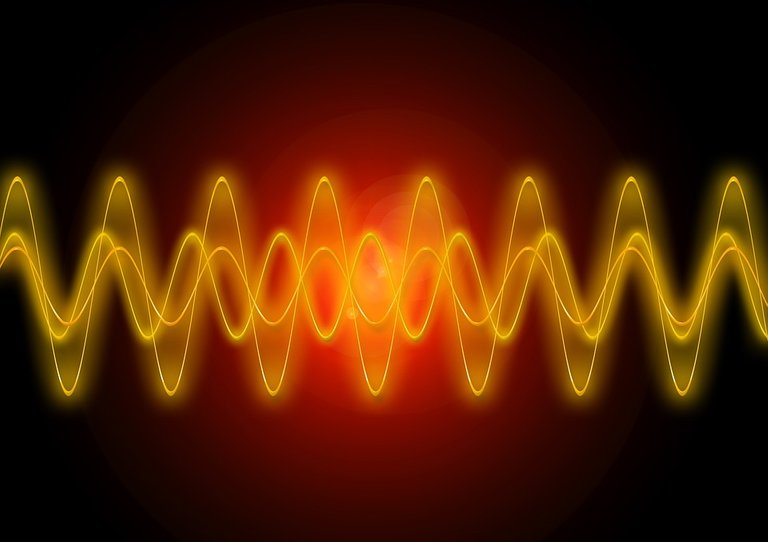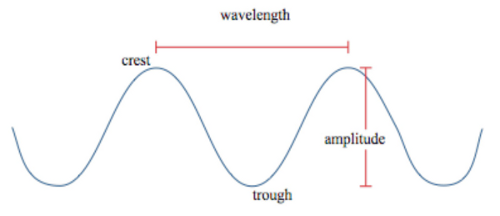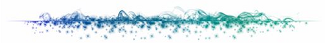# What are the frequencies? Means, Definition and Symbol

in #steemstem2 years ago (edited)

In general, frequency is a measure of how often something happens over a period of time. In science and technology, frequencies are measured in Hz (hertz).

With respect to the CPU, frequency refers to the processor's operational clock cycle per second. The frequency of most modern CPUs is measured in GHz (GigaHertz) or billions of cycles per second.

Frequency alternating electrical current is the number of oscillations.The term frequency can be referred to as an electromagnetic wave per second, such as a sound wave or light wave. The frequency of light determines its color, as seen by the human eye. Similarly, the frequency of sound determines its pitch, as heard by a human's ear.

Definition of frequency

Have you ever wondered why the sky is blue? Or, more than this point, why is anything colored? Your father's voice was deep, and your mother's voice was not so deep. Whether it is color, or notes on the piano, all of these things are explained by frequency.

Frequency is a property of a wave. We are surrounded by waves every day. Light is an electromagnetic wave, and the fan sound in your computer is a sound wave. A wave (wave) is a vibration that carries energy with it. The frequency of a waveform is the number of waves that pass through each second, and is measured in Hertz (Hz). For example, the frequency of a sound wave can be 450 Hz.

Frequency is the number of oscillations / oscillations per minute. It is used to define cycle processes such as rotation, oscillation, wave etc. The completion of a cycle process at particular intervals of time is known as frequency.

The SI unit of frequency is Hertz. The symbol λ represents this. A Hertz means that the wave completes one cycle in a second. The traditional unit for measuring cycle processes is revolution per second, which is equal to one hertz.Frequency is a parameter that explains the oscillation and vibration phenomena, such as mechanical vibrations, sound signals, light, frequency waves, etc. The term "period" represents the time required by the waveform for an oscillation, that is, it is inversely proportional to the frequency.

Frequency is the total number of oscillations per unit time. If we take the example of flash, the period is the time between two flash. And frequency is the total number of flash per second.

Relation Between Wave δ Frequency

Wave is a type of disturbance that is used to transfer information. Information is transferred as oscillations. Wave is the occurrence of period and wave frequency oscillations. The duration of the wave is the difference between the wave and the frequency of the wave is the number of waves per unit time.

Types of Frequency:

Frequencies are mainly classified into two categories.

1) Angular Frequency:

Angular Frequency refers to the number of revolutions at certain intervals of time. The unit of angular frequency is Hertz. The relationship between Frequency and Angular Frequency is expressed as-

ω = 2πf

Where ,, ω - angular frequency

2) Spatial Frequency

The frequency that depends on the spatial coordinate is known as the spatial frequency. It is inversely proportional to the wavelength. Spatial Frequency measures the characteristic of a structure that is periodic in space.

Relationship Between Frequency And Wavelength

The wavelength and frequency of light are closely related. High frequency, low wavelength. Because all light waves pass through a vacuum at the same speed, the number of waves passing through a given point in a second depends on the wavelength. The number, also known as the frequency, is larger for a short-wavelength than a long-wavelength.

The equation that relates the wavelength and frequency for electromagnetic waves:

λν = c

Where λ is the wavelength

ν is the frequency

And c is the speed of light

### [Ref 1](https://en.wikipedia.org/wiki/Frequency)

![image.png]()

#### @orion7Sort:

Everything is frequency, so mysterious that the ancient mystics knew this so long ago

Yeah the mystics must be knowing long ago but defining the frequency along with related parameters such as it's unit, phase, wave length etc. came much later, which we all study in nowadays books. Thanks for feedback @fenngen

2 years ago Reveal Comment

Thank you very much.